# 15-344/Classnotes for Tuesday September 15

We mostly went over Day One Handout today.

 Dror's notes above / Students' notes below

## Lecture Note for September 15

DEFINITION 1 Graph A graph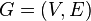$G = (V,E)$ is a set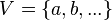$V = \{a,b,...\}$ (usually finite, "vertices") along with a set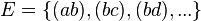$E = \{(ab),(bc),(bd),...\}$ ("edges") of unordered pairs of distinct elements of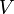$V$.

DEFINITION 2 Incident If an edge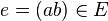$e = (ab)\in{E}$, we say that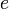$e$ is incident to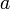$a$ and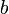$b$.

DEFINITION 3 N-valent In a graph$G = (V,E)$, a vertex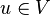$u \in{V}$ is called bivalent if it is incident to precisely two edges and n-valent if incident to precisely n edges, where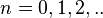$n = 0,1,2,..$.

DEFINITION 4 Edge Cover An edge cover for graph$G = (V,E)$ is a subset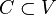$C\subset{V}$ such that every edge of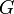$G$ incident to at least one vertex in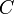$C$.

DEFINITION 5 Independent Let$G = (V,E)$ be a graph. A subset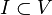$I\subset{V}$ is called independent if whenever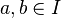$a,b\in{I}$, then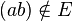$(ab)\notin{E}$.

THEOREM 1 Edge covers are complementary to independent sets. In other words,$C\subset{V}$ is an edge cover if and only if the complementary subset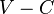$V-C$ is an independent set.

Proof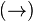$(\rightarrow)$ Assume$C$ is an edge cover. I assert that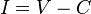$I = V-C$ is independent. Indeed, if$e=(ab)\in{E}$, then since$C$ is an edge cover, either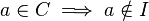$a\in{C} \implies a\notin{I}$ or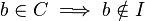$b\in{C} \implies b\notin{I}$, which implies$e$ does not connect any two elements of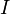$I$.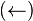$(\leftarrow)$ Assume$I=V-C$ is independent. Pick any edge$e = (ab)\in{E}$. As$I$ is independent,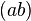$(ab)$ does not connect any two members of$I$. Hence, either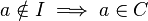$a\notin{I} \implies a\in{C}$ or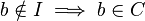$b\notin{I} \implies b\in{C}$, which implies$e$ is incident to an element of$C$. QED

DEFINITION 6 Matching is a subset of edges of a graph where no two edges share a common vertex. A matching is said to be perfect if all edges in a graph are matched.